Courses

# RD Sharma Solutions -Ex-20.1, Mensuration, Class 6, Maths Class 6 Notes | EduRev

## RD Sharma Solutions for Class 6 Mathematics

Created by: Abhishek Kapoor

## Class 6 : RD Sharma Solutions -Ex-20.1, Mensuration, Class 6, Maths Class 6 Notes | EduRev

The document RD Sharma Solutions -Ex-20.1, Mensuration, Class 6, Maths Class 6 Notes | EduRev is a part of the Class 6 Course RD Sharma Solutions for Class 6 Mathematics.
All you need of Class 6 at this link: Class 6

Question 1. Which of the following are closed curves? Which of them are simple?

(i)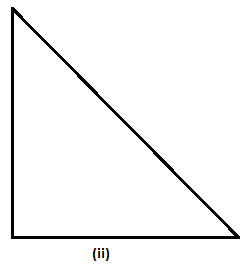(ii)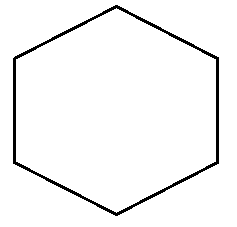(iii)

(iv)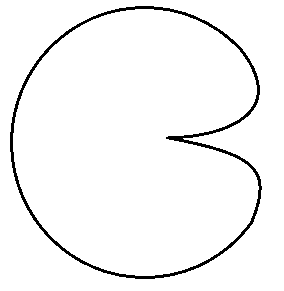(v)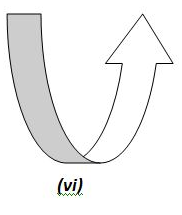Figure (ii), (iii), (iv), (vi), and (vii) are closed curves, whereas Figure (ii), (iii), (iv) and (vi) are simple closed curves.

Question 2. Define perimeter of a closed figure.

Answer: The length of the boundary of a closed figure is known as its permieter.

Question 3. Find the perimeter of each of the following shapes: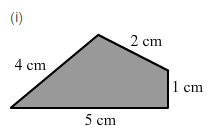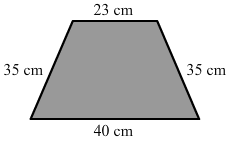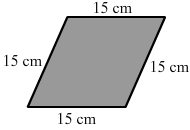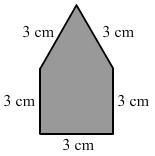Answer: Perimeter = Sum of lengths of all sides os a closed figure

(i) Perimeter = ( 4 + 2 + 1 + 5) cm = 12 cm

(ii) Perimeter = (23 + 35 + 40 + 35) cm = 133 cm

(iii) Perimeter = (15 + 15 + 15 + 15) cm = 60 cm

(iv) Perimeter = (3 + 3 + 3 + 3 + 3) cm = 15 cm

103 docs

,

,

,

,

,

,

,

,

,

,

,

,

,

,

,

,

,

,

,

,

,

,

,

,

,

,

,

,

,

,

;